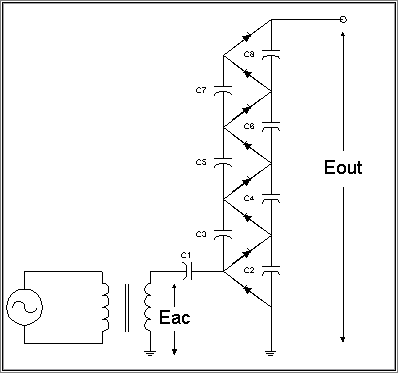## Cockroft Walton Voltage Multipliers

The classic multistage diode/capacitor voltage multipler, popularized by Cockroft and Walton, is probably the most popular means of generating high voltages at low currents at low cost. It is used in virtually every television set made to generate the 20-30 kV second anode accelerating voltage from a transformer putting out 10-15 kV pulses. It has the advantage of requiring relatively low cost components and being easy to insulate. It also inherently produces a series of stepped voltages which is useful in some forms of particle accelerators, and for biasing photomultipler tube dynodes.

The CW multiplier has the disadvantage of having very poor voltage regulation, that is, the voltage drops rapidly as a function the output current. In some applications, this is an advantage. The output V/I characteristic is roughly hyperbolic, so it serves well for charging capacitor banks to high voltages at roughly constant charging power. Furthermore, the ripple on the output, particularly at high loads, is quite high.

It is quite popular for relatively low powered particle accelerators for injecting into another accelerator, particularly for heavy ions. The high ripple means that there is a signficant energy spread in the ion beam, though, and for applications where low ripple is important at megavolt potentials, electrostatic systems like Van de Graaf and Pelletron machines are preferred.The output voltage (Eout) is nominally the input voltage (Eac) multiplied by the number of stages, 4 in the above diagram. However, in practice, the output is significantly lower, particularly with a large number of stages.

### Regulation and ripple calculations

The voltage drop under load can be calculated as:

```Edrop = I1/ (f*C) * (2 /3*n^3 + n^2/2- n/6)```

where:
C is the stage capacitance
f is the AC frequency
n is the number of stages.

The ripple voltage, in the case where all stage capacitances (C1 through C(2*n)) may be calculated from:
```Eripple = Iload/(f * C)*n*(n+1)/2```

As you can see from this equation, the ripple grows quite rapidly as the number of stages increases (as n squared, in fact). A common modification to the design is to make the stage capacitances larger at the bottom, with C1 & C2 = nC, C3 & C4= (n-1)C, and so forth. In this case, the ripple is:
`Eripple = Iload/(f*C)`

For large values of n (>= 5), the n2/2 and n/6 terms in the voltage drop equation become small compared to the 2/3n3. Differentiating the drop equation with respect to the number of stages gives an equation for the optimum number of stages (for the equal valued capacitor design:

```Noptimum = SQRT( Vmax * f * C/Iload)```

Increasing the frequency can dramatically reduce the ripple, and the voltage drop under load.

#### A modification to the basic Cockroft Walton multipler

In some applications, an addtional capacitor stack is connected to the output capacitor stack in the above design. This is particularly popular in electrostatic accelerator applications and high voltage x-ray systems, where low ripple desired.

### Another modification to the basic Cockroft Walton design

A modification to the classic CW multiplier, popularlized by XXX, uses two charging stacks driven by out of phase input voltages. This is particularly useful when the charging stack capacitors are significantly smaller than the output filter capacitors. The XXX modification is illustrated in the following figure.Copyright 19987, Jim Lux / cw1.htm / 3 May 1998 / Back to HV Home / Back to home page / Mail to Jim